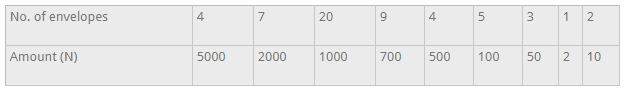mycollegehive
Donation during the launching of church project were in seal...
math waec-2018 ssce-exam-2018 waec-mathematics-20180Donation during the launching of church project were in sealed enveloped . The table show the distribution of the amount of money in the envelopeHow much was the total donation?

A. 26,792.00

B. 26,972.00

C. 62,792.00

D. 62,972.00

72 viewsShareFollowUniversity of Lagos Nigeria
19 May 2020University of Benin Nigeria
31 August 202004 envelopes at N5,000 each = 4 x 5000 = 20,000

7 envelopes at N2,000 each = 7 x 2000 = 14,000

20 envelopes at N1,000 each = 20 x 1000 = 20,000

9 envelopes at N700each = 9 x 700 = 6,300

4 envelopes at N500 each = 4 x 500 = 2,000

5 envelopes at N100 each = 5 x 100 = 500

3 envelopes at N50 each = 3 x 50 = 150

1 envelopes at N2 each = 1 x 2 = 2

2 envelopes at N10 each = 2 x 10 = 20

Total = 20000 + 14000 + 20000 + 6300 + 2000 + 500 + 150 + 2 + 20

= 62, 972

Ans DShare

### Groups

How to insert math formulas/equations### Related Tags

math

15 followers

1261 questionswaec-2018

1 followers

29 questionsssce-exam-2018

1 followers

29 questionswaec-mathematics-2018

1 followers

28 questions